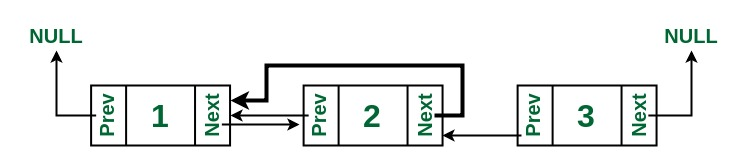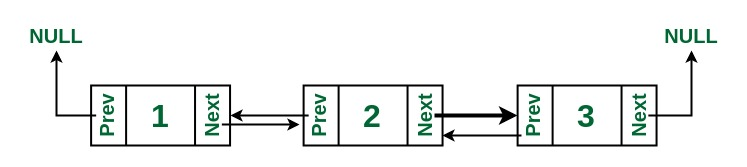# Correct the Random Pointer in Doubly Linked List

Given a doubly linked list having exactly one of the node pointing to a random node in the list, the task is to correct this random pointer in the doubly linked list, such that it points to the expected node.

Examples:

Input:Output:Explanation: 2’s next pointer has been corrected to point to 3. Earlier it was pointing to 1, which was incorrect.

Approach: This can be achieved by simply iterating the list and checking the individual pointers.

Below is the implementation for the above approach:

## C++

 `// C++ program to Correct ` `// the Random Pointer in Doubly Linked List ` `#include ` `using` `namespace` `std; ` ` `  `// Node of a doubly linked list ` `struct` `node { ` `    ``int` `data; ` ` `  `    ``// Pointer to next node in DLL ` `    ``node* next; ` ` `  `    ``// Pointer to next node in DLL ` `    ``node* prev; ` `}; ` ` `  `// Funciton to allocate node ` `node* newNode(``int` `data) ` `{ ` `    ``node* temp = ``new` `node; ` `    ``temp->data = data; ` `    ``temp->next = temp->prev = NULL; ` `    ``return` `temp; ` `} ` ` `  `// Function to correct the random pointer ` `void` `correctPointer(node*& head) ` `{ ` ` `  `    ``if` `(!head) ` `        ``return``; ` ` `  `    ``node* temp = head; ` ` `  `    ``// if head->next's previous is not ` `    ``// pointing to head itself, ` `    ``// change it. ` `    ``if` `(head->next && head->next->prev != head) { ` ` `  `        ``head->next->prev = head; ` `        ``return``; ` `    ``} ` ` `  `    ``// If head's previous pointer is incorrect, ` `    ``// change it. ` `    ``if` `(head->prev != NULL) { ` ` `  `        ``head->prev = NULL; ` `        ``return``; ` `    ``} ` ` `  `    ``// Else check for remaining nodes. ` `    ``temp = temp->next; ` `    ``while` `(temp) { ` ` `  `        ``// If node->next's previous pointer is ` `        ``// incorrect, change it. ` `        ``if` `(temp->next && temp->next->prev != temp) { ` ` `  `            ``temp->next->prev = temp; ` `            ``return``; ` `        ``} ` ` `  `        ``// Else If node->prev's next pointer is  ` `        ``// incorrect, change it. ` `        ``else` `if` `(temp->prev && temp->prev->next != temp) { ` ` `  `            ``temp->prev->next = temp; ` `            ``return``; ` `        ``} ` ` `  `        ``// Else iterate on remaining. ` `        ``temp = temp->next; ` `    ``} ` `} ` ` `  `// Function to print the DLL ` `void` `printList(node* head) ` `{ ` `    ``node* temp = head; ` ` `  `    ``while` `(temp) { ` ` `  `        ``cout << temp->data << ``" ("``; ` ` `  `        ``// If prev pointer is null, print -1. ` `        ``cout << (temp->prev ` `                     ``? temp->prev->data ` `                     ``: -1) ` `             ``<< ``") "``; ` ` `  `        ``temp = temp->next; ` `    ``} ` `    ``cout << endl; ` `} ` ` `  `int` `main() ` `{ ` ` `  `    ``// Creating a DLL ` `    ``node* head = newNode(1); ` `    ``head->next = newNode(2); ` `    ``head->next->prev = head; ` `    ``head->next->next = newNode(3); ` `    ``head->next->next->prev = head; ` `    ``head->next->next->next = newNode(4); ` `    ``head->next->next->next->prev = head->next->next; ` ` `  `    ``cout << ``"\nIncorrect Linked List: "``; ` `    ``printList(head); ` ` `  `    ``correctPointer(head); ` ` `  `    ``cout << ``"\nCorrected Linked List: "``; ` `    ``printList(head); ` ` `  `    ``return` `0; ` `} `

## Java

 `// Java program to Correct ` `// the Random Pointer in Doubly Linked List ` `class` `GFG  ` `{ ` ` `  `// Node of a doubly linked list ` `static` `class` `node  ` `{ ` `    ``int` `data; ` ` `  `    ``// Pointer to next node in DLL ` `    ``node next; ` ` `  `    ``// Pointer to next node in DLL ` `    ``node prev; ` `}; ` ` `  `// Funciton to allocate node ` `static` `node newNode(``int` `data) ` `{ ` `    ``node temp = ``new` `node(); ` `    ``temp.data = data; ` `    ``temp.next = temp.prev = ``null``; ` `    ``return` `temp; ` `} ` ` `  `// Function to correct the random pointer ` `static` `void` `correctPointer(node head) ` `{ ` `    ``if` `(head == ``null``) ` `        ``return``; ` ` `  `    ``node temp = head; ` ` `  `    ``// if head->next's previous is not ` `    ``// pointing to head itself, ` `    ``// change it. ` `    ``if` `(head.next != ``null` `&&  ` `        ``head.next.prev != head)  ` `    ``{ ` `        ``head.next.prev = head; ` `        ``return``; ` `    ``} ` ` `  `    ``// If head's previous pointer is incorrect, ` `    ``// change it. ` `    ``if` `(head.prev != ``null``)  ` `    ``{ ` `        ``head.prev = ``null``; ` `        ``return``; ` `    ``} ` ` `  `    ``// Else check for remaining nodes. ` `    ``temp = temp.next; ` `    ``while` `(temp != ``null``)  ` `    ``{ ` ` `  `        ``// If node.next's previous pointer is ` `        ``// incorrect, change it. ` `        ``if` `(temp.next != ``null` `&&  ` `            ``temp.next.prev != temp)  ` `        ``{ ` `            ``temp.next.prev = temp; ` `            ``return``; ` `        ``} ` ` `  `        ``// Else If node.prev's next pointer is  ` `        ``// incorrect, change it. ` `        ``else` `if` `(temp.prev != ``null` `&& ` `                 ``temp.prev.next != temp)  ` `        ``{ ` `            ``temp.prev.next = temp; ` `            ``return``; ` `        ``} ` `        ``System.out.print(``""``); ` `         `  `        ``// Else iterate on remaining. ` `        ``temp = temp.next; ` `    ``} ` `} ` ` `  `// Function to print the DLL ` `static` `void` `printList(node head) ` `{ ` `    ``node temp = head; ` ` `  `    ``while` `(temp != ``null``)  ` `    ``{ ` ` `  `        ``System.out.print(temp.data + ``" ("``); ` ` `  `        ``// If prev pointer is null, print -1. ` `        ``System.out.print((temp.prev != ``null` `? ` `                          ``temp.prev.data: -``1``) + ``") "``); ` ` `  `        ``temp = temp.next; ` `    ``} ` `    ``System.out.print(``"\n"``); ` `} ` ` `  `// Driver Code ` `public` `static` `void` `main(String[] args) ` `{ ` `    ``// Creating a DLL ` `    ``node head = newNode(``1``); ` `    ``head.next = newNode(``2``); ` `    ``head.next.prev = head; ` `    ``head.next.next = newNode(``3``); ` `    ``head.next.next.prev = head; ` `    ``head.next.next.next = newNode(``4``); ` `    ``head.next.next.next.prev = head.next.next; ` ` `  `    ``System.out.print(``"\nIncorrect Linked List: "``); ` `    ``printList(head); ` ` `  `    ``correctPointer(head); ` ` `  `    ``System.out.print(``"\nCorrected Linked List: "``); ` `    ``printList(head); ` `} ` `} ` ` `  `// This code is contributed by Princi Singh `

## Python3

 `# Python3 program to Correct the ` `# Random Pointer in Doubly Linked List ` ` `  `class` `Node: ` `     `  `    ``def` `__init__(``self``, data): ` `        ``self``.data ``=` `data ` `        ``self``.prev ``=` `None` `        ``self``.``next` `=` `None` ` `  `# Function to correct the random pointer ` `def` `correctPointer(head): ` ` `  `    ``if` `head ``=``=` `None``: ` `        ``return` ` `  `    ``temp ``=` `head ` ` `  `    ``# if head.next's previous is not ` `    ``# pointing to head itself, change it. ` `    ``if` `(head.``next` `!``=` `None` `and`  `        ``head.``next``.prev !``=` `head):  ` ` `  `        ``head.``next``.prev ``=` `head ` `        ``return` `     `  `    ``# If head's previous pointer is  ` `    ``# incorrect, change it. ` `    ``if` `head.prev !``=` `None``:  ` ` `  `        ``head.prev ``=` `None` `        ``return` `     `  `    ``# Else check for remaining nodes. ` `    ``temp ``=` `temp.``next` `    ``while` `temp !``=` `None``:  ` ` `  `        ``# If node.next's previous pointer  ` `        ``# is incorrect, change it. ` `        ``if` `(temp.``next` `!``=` `None` `and`  `            ``temp.``next``.prev !``=` `temp):  ` ` `  `            ``temp.``next``.prev ``=` `temp ` `            ``return` `         `  `        ``# Else If node.prev's next pointer  ` `        ``# is incorrect, change it. ` `        ``elif` `(temp.prev !``=` `None` `and`  `              ``temp.prev.``next` `!``=` `temp):  ` ` `  `            ``temp.prev.``next` `=` `temp ` `            ``return` `         `  `        ``# Else iterate on remaining. ` `        ``temp ``=` `temp.``next` `     `  `# Function to print the DLL ` `def` `printList(head): ` ` `  `    ``temp ``=` `head ` ` `  `    ``while` `temp !``=` `None``: ` ` `  `        ``print``(temp.data, ``"("``, end ``=` `"") ` ` `  `        ``# If prev pointer is null, print -1. ` `        ``if` `temp.prev ``=``=` `None``: ` `            ``print``(``-``1``, end ``=` `") "``) ` `        ``else``: ` `            ``print``(temp.prev.data, end ``=` `") "``) ` `         `  `        ``temp ``=` `temp.``next` `     `  `    ``print``() ` ` `  `# Driver Code ` `if` `__name__ ``=``=` `"__main__"``: ` ` `  `    ``# Creating a DLL ` `    ``head ``=` `Node(``1``) ` `    ``head.``next` `=` `Node(``2``) ` `    ``head.``next``.prev ``=` `head ` `    ``head.``next``.``next` `=` `Node(``3``) ` `    ``head.``next``.``next``.prev ``=` `head ` `    ``head.``next``.``next``.``next` `=` `Node(``4``) ` `    ``head.``next``.``next``.``next``.prev ``=` `head.``next``.``next` ` `  `    ``print``(``"Incorrect Linked List:"``,  ` `                         ``end ``=` `" "``) ` `    ``printList(head) ` ` `  `    ``correctPointer(head) ` ` `  `    ``print``(``"\nCorrected Linked List:"``, ` `                           ``end ``=` `" "``) ` `    ``printList(head) ` ` `  `# This code is contributed  ` `# by Rituraj Jain `

## C#

 `// C# program to Correct the  ` `// Random Pointer in Doubly Linked List ` `using` `System; ` `class` `GFG  ` `{ ` ` `  `// Node of a doubly linked list ` `class` `node  ` `{ ` `    ``public` `int` `data; ` ` `  `    ``// Pointer to next node in DLL ` `    ``public` `node next; ` ` `  `    ``// Pointer to next node in DLL ` `    ``public` `node prev; ` `}; ` ` `  `// Funciton to allocate node ` `static` `node newNode(``int` `data) ` `{ ` `    ``node temp = ``new` `node(); ` `    ``temp.data = data; ` `    ``temp.next = temp.prev = ``null``; ` `    ``return` `temp; ` `} ` ` `  `// Function to correct the random pointer ` `static` `void` `correctPointer(node head) ` `{ ` `    ``if` `(head == ``null``) ` `        ``return``; ` ` `  `    ``node temp = head; ` ` `  `    ``// if head->next's previous is not ` `    ``// pointing to head itself, ` `    ``// change it. ` `    ``if` `(head.next != ``null` `&&  ` `        ``head.next.prev != head)  ` `    ``{ ` `        ``head.next.prev = head; ` `        ``return``; ` `    ``} ` ` `  `    ``// If head's previous pointer is incorrect, ` `    ``// change it. ` `    ``if` `(head.prev != ``null``)  ` `    ``{ ` `        ``head.prev = ``null``; ` `        ``return``; ` `    ``} ` ` `  `    ``// Else check for remaining nodes. ` `    ``temp = temp.next; ` `    ``while` `(temp != ``null``)  ` `    ``{ ` ` `  `        ``// If node.next's previous pointer is ` `        ``// incorrect, change it. ` `        ``if` `(temp.next != ``null` `&&  ` `            ``temp.next.prev != temp)  ` `        ``{ ` `            ``temp.next.prev = temp; ` `            ``return``; ` `        ``} ` ` `  `        ``// Else If node.prev's next pointer  ` `        ``// is incorrect, change it. ` `        ``else` `if` `(temp.prev != ``null` `&& ` `                 ``temp.prev.next != temp)  ` `        ``{ ` `            ``temp.prev.next = temp; ` `            ``return``; ` `        ``} ` `        ``Console.Write(``""``); ` `         `  `        ``// Else iterate on remaining. ` `        ``temp = temp.next; ` `    ``} ` `} ` ` `  `// Function to print the DLL ` `static` `void` `printList(node head) ` `{ ` `    ``node temp = head; ` ` `  `    ``while` `(temp != ``null``)  ` `    ``{ ` `        ``Console.Write(temp.data + ``" ("``); ` ` `  `        ``// If prev pointer is null, print -1. ` `        ``Console.Write((temp.prev != ``null` `? ` `                       ``temp.prev.data: -1) + ``") "``); ` ` `  `        ``temp = temp.next; ` `    ``} ` `    ``Console.Write(``"\n"``); ` `} ` ` `  `// Driver Code ` `public` `static` `void` `Main(String[] args) ` `{ ` `    ``// Creating a DLL ` `    ``node head = newNode(1); ` `    ``head.next = newNode(2); ` `    ``head.next.prev = head; ` `    ``head.next.next = newNode(3); ` `    ``head.next.next.prev = head; ` `    ``head.next.next.next = newNode(4); ` `    ``head.next.next.next.prev = head.next.next; ` ` `  `    ``Console.Write(``"\nIncorrect Linked List: "``); ` `    ``printList(head); ` ` `  `    ``correctPointer(head); ` ` `  `    ``Console.Write(``"\nCorrected Linked List: "``); ` `    ``printList(head); ` `} ` `} ` ` `  `// This code is contributed by PrinciRaj1992 `

Output:

```Incorrect Linked List: 1 (-1) 2 (1) 3 (1) 4 (3)

Corrected Linked List: 1 (-1) 2 (1) 3 (2) 4 (3)
```

My Personal Notes arrow_drop_upA Coding Enthusiast Rails Developer

If you like GeeksforGeeks and would like to contribute, you can also write an article using contribute.geeksforgeeks.org or mail your article to contribute@geeksforgeeks.org. See your article appearing on the GeeksforGeeks main page and help other Geeks.

Please Improve this article if you find anything incorrect by clicking on the "Improve Article" button below.

Article Tags :
Practice Tags :

Be the First to upvote.

Please write to us at contribute@geeksforgeeks.org to report any issue with the above content.